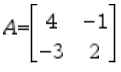# Knowledge Base

## Solution 33891: Calculating the Determinant of a Matrix Using a TI-36X Pro.

### How do I calculate the determinant of a matrix using a TI-36X Pro?

The instructions below will demonstrate how to find the determinant of a matrix using a TI-36X Pro.

For Example: What is the determinant of matrix A?1) Press [2nd] [matrix].
2) Scroll to EDIT and press [enter].
3) Select 2 for ROWS and 2 for COLUMNS.
4) Scroll to OK and press [enter].
5) Press  [enter] [(-)]  [enter] [(-)]  enter]  [enter].
6) Exit the matrix editor by selecting [2nd] [quit].

To find the Determinant using the function:

7) Press [2nd] [matrix].
8) Scroll to MATH.
9) Press 1: Determinant.
10) Press [2nd] [matrix].
11) Under NAMES, press 1: [A] 2x2.
12) Press [enter] to display the answer of 5.